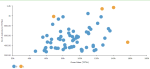# SMILE.OUTLIERS(std_deviation_X, columns)

Calculates outliers using the Mahalanobis distance measures. In statistics, Mahalanobis distance is based on correlations between variables by which different patterns can be identified and analyzed. It is a useful way of determining similarity of an unknown sample set to a known one. It differs from the Euclidean distance in that it takes into account the correlations of the data set and is not dependent on the scale of measurements.

###### Parameters
• std_deviation_X – Standard deviation threshold of Mahalanobis distance, after which data point is considered as an outlier; integer (for example, 3).

• columns – Dataset columns or custom calculations.

Example: SMILE.OUTLIERS(3, sum([No of customers]), sum([Gross Sales])) used as a calculation for the Color field of the Scatterplot visualization.###### Input data
• Numeric variables
• Without missing values
• Size of input data is not limited
###### Result
• Column of integer values 0 or 1, where 1 is outlier and 0 is inlier.
###### Key usage points
• It is a multivariate outlier detection method, so multiple variables are allowed.
• Only numeric (continuous) variables are allowed
• Inappropriate for ordinal data
• Calculation of sample covariance matrix makes it self-sensitive to outliers

For the whole list of algorithms, see Data science built-in algorithms.